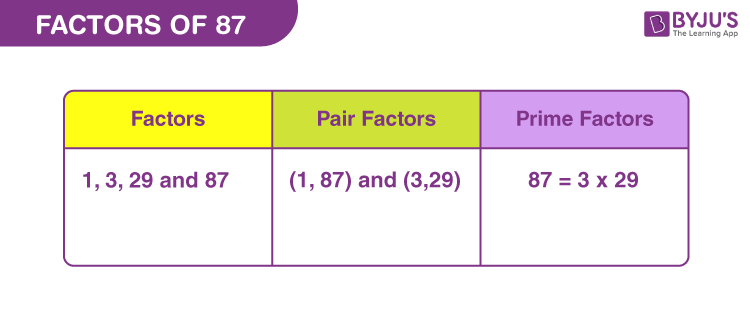# Factors of 87

In Maths, factors of 87 are the natural numbers that divide the original number into equal parts. The product of two natural numbers results in 87, hence they become the factors of eighty-seven. 87 is an odd composite number, thus has more than two factors.

Let us find the factors of 87 along with pair factors and prime factors, using simple division and prime factorisation methods, respectively.## How to Find Factors of 87?

The factor of 87 breaks the original number into equal numbers of parts. Therefore, there will be no remainder left after division. Let us see how to find the factors of the real number 87.

87 ÷ 1 = 87 [Remainder = 0]

87 ÷ 3 = 29 [Remainder = 0]

87 ÷ 29 = 3 [Remainder = 0]

87 ÷ 87 = 1 [Remainder = 0]

Thus, the factors of 87 are 1, 3, 29 and 87.

## Pair Factors of 87

The pair factors of 87 are formed when two integers are multiplied together. Therefore, we can observe, there are only two such pairs of numbers that can generate the original number, on multiplication.

1 × 87 = 87

3 × 29 = 87

Therefore, the pair factors are (1, 87) and (3, 29).

Now, if we consider the negative integers as a pair factors of 87, then we get;

-1 × -87 = 87

-3 × -29 = 87

As we can see, from the above multiplication, two negative integers on multiplication with each other results in the original number. Therefore, the negative pair factors are (-1, -87) and (-3, -29).

## Prime Factorisation of 87

By prime factorisation of 87, we can determine the prime numbers that can uniformly divide the original number. Thus, in this case, we will be dividing 87, only by the prime numbers.

Before we start evaluating the prime factors, let us see what are the primes between 1 and 87

List of prime numbers (Between 1 and 87) :

 2, 3, 5, 7, 11, 13, 17, 19, 23, 29, 31, 37, 41, 43, 47, 53, 59, 61, 67, 71, 73, 79, 83

Follow the steps below to find the prime factors.

Step 1: The smallest prime number by which 87 is divisible, is 3.

87/3 = 29

Step 2: Now, if we see, from the list of primes, 29 itself is a prime number. Therefore, it is divisible by itself or 1. Since, 1 is not a prime number, therefore we will ignore it.

29/29 = 1

Therefore, the prime factors of 87 are 3 and 29.

 Prime factorisation of 87 = 3 x 29 Exponential Form = 31 x 291

## Solved Examples

Q.1: Find the sum of all the factors of 87. Also, find if the sum is a prime number or composite number.

Solution: The factors of 87 are 1, 3, 29 and 87 .

Sum = 1 + 3 + 29 + 87 = 120

Therefore, 120 is the required sum.

120 is a composite number, because it has more than two factors.

Factors of 120 are 1, 2, 3, 4, 5, 6, 8, 10, 12, 15, 20, 24, 30, 40, 60, 120.

Q.2: What is the greatest common factor of 90 and 87?

Answer: Let us first write the factors of both the numbers.

Factors of 87 → 1, 3, 29, 87

Factors of 90 → 1, 2, 3, 5, 6, 9, 10, 15, 18, 30, 45, 90

Therefore, greatest common factor (GCF) of 87 and 90 = 3

Q.3: What are the common factors of 85, 86 and 87?

85 → 1, 5, 17, 85

86 → 1, 2, 43, 86

87 → 1, 2, 43, 87

Therefore, the common factor is only 1.

### Practice Problems

1. Find the average value of factors of 87.
2. Find if 87 and 82 are co-prime numbers or not.
3. John has 81 balls in the red basket and 87 balls in the blue basket. What is the maximum number of balls he can distribute equally in each basket?
4. What is the GCF of 81 and 87?

## Frequently Asked Questions on Factors of 87

### What are the factors of 87?

There are a total of four factors of 87. These factors are 1, 3, 29 and 87.

### What is the prime factor form of 87?

The prime factorisation of 87 is: 3 x 29.

### Is 87 a prime number? If not why?

87 is not a prime number but a composite number because it exceeds the fact of having two factors.

### What are the multiples of 87?

The first ten multiples of 87 are 87, 174, 261, 348, 435, 522, 609, 696, 783 and 870.

### Is 87 a perfect square?

87 is not a perfect square, because the square of no natural number results in 87.